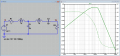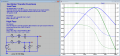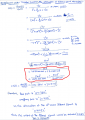# How to calculate the attenuation of filtered signal?

#### pinkyponky

Joined Nov 28, 2019
195
Last edited:

#### MrAl

Joined Jun 17, 2014
8,556
Hi,

My first question is that the 2-pole second order passive RLC low pass filter is a 4th order filter?, as shown below. And also, what is the formula for Attenuation and how to calculate the Attenuation of this filtered signal (which is appear at the output of L2) ?.

View attachment 251239
The 'formula' is really the transfer function sometime denoted by "T(s)". It's really just an AC network analysis.
Do you know how to calculate the transfer function?
If you know how to analyze the network with all resistors, then just change any C or L into it's complex impedance and go from there using complex number analysis.
Just to note the three impedances you need to work with...
Resistor R => R
Capacitor C => -j*w*C (or just 1/(s*C))
Inductor, L => j*w*L (or just s*L)
(and for L and C w=2*pi*f)
AC voltage source can be just the peak value or RMS value.
Note R does not change but L and C do.
The math is the same the only difference is you will be using complex numbers like 3+5*j.
If you use the forms 1/(s*C) and s*L then the only difference is you will be using algebra as well as some numerical calculations.
Example of RC series circuit also in series with an AC voltage source with the output from across the C:
Vout(s)/Vin(s)=T(s)=(1/(s*C))/(1/(s*C)+R) and simplified: T(s)=1/(s*C*R+1)
and then you can make s=j*w if you like and then change the frequency.
That last expression turns into T(s)1/(j*w*C*R+1) and with f=100Hz we get
T(s)=1/(j*2*pi*100*R*C+1)=1/(j*200*pi*R*C+1)
You can then convert that into an amplitude and phase angle or just amplitude if that is all you need.
Using f=100 and R=2 and C=3 and input of 1 volt peak we would end up with:
7.0361928e-8-j*2.6525822e-4 to 8 significant figures in units of peak voltage,
so the real part is 7.0361928e-8 and the imaginary part is -j*2.6525822e-4.
We can then calculate the AC peak amplitude by finding the norm of that, and get:
2.65258e-4 volts peak (amplitude)
and the phase angle:
So the output in this case is small and phase angle nearly -90 degrees. Other frequencies will produce different amplitudes and phase angles.

Last edited:

#### Papabravo

Joined Feb 24, 2006
17,296
Hi,

My first question is that the 2-pole second order passive RLC low pass filter is a 4th order filter?, as shown below. And also, what is the formula for Attenuation and how to calculate the Attenuation of this filtered signal (which is appear at the output of L2) ?.

View attachment 251239
Locate R2 properly by placing it in parallel with C2. In your schematic the low frequency response will be down much further than you expect because there is no DC path to ground.

It is a 4th order filter.

#### pinkyponky

Joined Nov 28, 2019
195
Hi MrAl,

My first question is that the 2-pole second order passive RLC low pass filter is a 4th order filter?, as shown above.
Is this { T(s)=1/(s*C*R+1) } transfer function of 4th order RLC filter?. If not, please can you post me the formula for 4th order RLC filter?
What is the units of these R=2 and C=3 values?. How you chosen these values?.

Last edited:

#### Papabravo

Joined Feb 24, 2006
17,296
Hi MrAl,

My first question is that the 2-pole second order passive RLC low pass filter is a 4th order filter?, as shown above.
Is this { T(s)=1/(s*C*R+1) } transfer function of 4th order RLC filter?. If not, please can you post me the formula for 4th order RLC filter?
What is the units of these R=2 and C=3 values?. How you chosen these values?.
You have cascaded two 2nd order filters which makes a 4th order filter. You should be able to work out the two different 2nd order responses. The combined response is the product of the two. There is a problem however and that problem is the mismatch between the output impedance of the 1st filter does not match the input impedance of the 2nd filter so there are additional losses.

#### pinkyponky

Joined Nov 28, 2019
195
You have cascaded two 2nd order filters which makes a 4th order filter. You should be able to work out the two different 2nd order responses. The combined response is the product of the two. There is a problem however and that problem is the mismatch between the output impedance of the 1st filter does not match the input impedance of the 2nd filter so there are additional losses.
Hi Papabravo,

The problems will see later, first I would like to know how to do the calculations the attenuation of filtered signals at each stage. So please could you answer the questions which are listed below:
Is this { T(s)=1/(s*C*R+1) } transfer function of 4th order RLC filter?. If not, please can you post me the formula for 4th order RLC filter?
What is the units of these R=2 and C=3 values?. How you chosen these values?.

#### Papabravo

Joined Feb 24, 2006
17,296
Hi Papabravo,

The problems will see later, first I would like to know how to do the calculations the attenuation of filtered signals at each stage. So please could you answer the questions which are listed below:
Is this { T(s)=1/(s*C*R+1) } transfer function of 4th order RLC filter?. If not, please can you post me the formula for 4th order RLC filter?
What is the units of these R=2 and C=3 values?. How you chosen these values?.
No T(s)=1/(sRC+1) is the transfer function for a 1st order (one reactive element) filter. An example of a fourth order filter with the two 2nd order factors shown would be something like:

$T(s)\;=\;\frac{105}{s^4+10s^3+45s+105s+105}\;=\;\frac{9.14013\cdot 11.4878}{(s^2+5.74292s+9.14013)(s^2+4.20758s+11.4878)}$

In case you are wondering this happens to be the transfer function for a 4th order Bessel Filter. It has a lowpass characteristic. A Bessel filter is derived in such a way that the group delay stays nearly constant in the passband.

As to the method of derivation for a transfer function you do it the same way you would do if for a network of resistors. Node equations and loop equations except now you have to do it with complex impedances.

On to your problem. You did not indicate that you were able to run the simulation. What you drew was a pretty crappy bandpass filter as you can see. I have rearranged what you drew slightly so there is a path from the voltage source to ground at DC (0 frequency). The minimum attenuation in the passband is 6 dB due to the 1Ω source impedance and the 1Ω load impedance. After that you can clearly see that the two LC sections are dramatically affecting each other. this is one reason why people do not design random combinations of components. They start with a proper set of requirements so they know what done looks like.Last edited:

#### pinkyponky

Joined Nov 28, 2019
195
$T(s)\;=\;\frac{105}{s^4+10s^3+45s+105s+105}\;=\;\frac{105}{(s^2+5.74292s+9.14013)(s^2+4.20758s+11..4878)}$
Hi Papa,

Where those values come from which are in the above question?.

I need help from you that, I want have a 2nd order and 4th order equations, and also the attenuation value to be calculated from the above simulation circuit (from the post 1). Please don't make me complex. I'm also expecting from you that the calculations would be more readable and understandable.

Last edited:

#### Papabravo

Joined Feb 24, 2006
17,296
Hi Papa,

Where those values come from which are in the above question?.

I need help from you that, I want have a 2nd order and 4th order equations, and also the attenuation value to be calculated from the above simulation circuit (from the post 1). Please don't make me complex. I'm also expecting from you that the calculations would be more readable and understandable.

They come from the condition that describes the transfer function for a Bessel Filter. The condition is that the phase be linear with a negative slope, the magnitude will be constant, and then the group delay will be constant.

See VanValkenburg, M.E., Analog Filter Design, 1982, pp. 279-289

As for your circuit, you should divide it into two separate and simpler problems, each of 2nd order. These simpler problems each have a single loop and their derivations should be straightforward. When you solve the two simpler problems, you multiply the two transfer functions together. This gives you a 4th order transfer function, which assumes that the second section does not present an appreciable load to the first section. It does do that so you have to work a bit harder to get the result you are looking for. The reason it is not worth doing for either educational or practical reasons is because designs for BETTER bandpass filters are know and can be computed directly.

EDIT: Here is some help on computing the second order equations you needLast edited:

#### pinkyponky

Joined Nov 28, 2019
195
Hi Again,

I was totally confused. Why do you simulated the different circuit?. Just provide me the formula to calculate the attenuation of 2nd order series RLC filter?. If possible, please also do the calculation in order to find the attenuation value at the end of the each 2nd order filter which is shown in the first post.

#### Papabravo

Joined Feb 24, 2006
17,296
Hi Again,

I was totally confused. Why do you simulated the different circuit?. Just provide me the formula to calculate the attenuation of 2nd order series RLC filter?. If possible, please also do the calculation in order to find the attenuation value at the end of the each 2nd order filter which is shown in the first post.
I've simulated both of the circuits from your original post separately to guide you in how to derive their individual transfer functions. I've show you how you can derive the 2nd order transfer function for each circuit. To find the 4th order transfer function you need multiply the two 2nd order transfer functions together. The algebra does require some care. You need to make some effort here.

R1, L1, C1, RL1 form the 2nd order lowpass filter. It's response is the green trace. Notice how flat the response is up to about 100 kHz.

R2, L2, C2, and RL2 form the 2nd order highpass filter. It's response is the blue trace. Notice that the response of this filter is NOT very flat above 100 kHz.

Refer back to the response in post #7 and look at that response. It is the sum of the responses in post #9
This is why it is hardly worth the effort to analyze. You can grind it out if you wish, but I'll take a pass.

Last edited:

#### The Electrician

Joined Oct 9, 2007
2,866
pinkyponky, is this homework? If it's not, why have you posted it in the homework forum?

#### pinkyponky

Joined Nov 28, 2019
195
I've simulated both of the circuits from your original post separately to guide you in how to derive their individual transfer functions. I've show you how you can derive the 2nd order transfer function for each circuit. To find the 4th order transfer function you need multiply the two 2nd order transfer functions together. The algebra does require some care. You need to make some effort here.

R1, L1, C1, RL1 form the 2nd order lowpass filter. It's response is the green trace. Notice how flat the response is up to about 100 kHz.

R2, L2, C2, and RL2 form the 2nd order highpass filter. It's response is the blue trace. Notice that the response of this filter is NOT very flat above 100 kHz.

Refer back to the response in post #7 and look at that response. It is the sum of the responses in post #9
This is why it is hardly worth the effort to analyze. You can grind it out if you wish, but I'll take a pass.
Hi again,

There is no high pass filter in my circuit (posted in #1), I don't want simulations, I want to know the value of the attenuation by doing the mathematical calculation. Just I want to have a value of attenuation after 2nd order filter and 4th order filter. And also I want to have fully mathematical calculation with formula, how the attenuation value is calculated?. That's it. I hope you understand my requirement.

#### pinkyponky

Joined Nov 28, 2019
195
pinkyponky, is this homework? If it's not, why have you posted it in the homework forum?
Hi,

Yes, it is a homework. Please could you help me.

#### The Electrician

Joined Oct 9, 2007
2,866
Hi again,

There is no high pass filter in my circuit (posted in #1), I don't want simulations, I want to know the value of the attenuation by doing the mathematical calculation. Just I want to have a value of attenuation after 2nd order filter and 4th order filter. And also I want to have fully mathematical calculation with formula, how the attenuation value is calculated?. That's it. I hope you understand my requirement.
This is the homework forum. You can't just post your "requirements" and expect somebody to do the work for you.

You need to learn to derive the transfer function for circuits like yours. Have a look on youtube:

There are other videos dealing with the same topic.

#### Papabravo

Joined Feb 24, 2006
17,296
Hi again,

There is no high pass filter in my circuit (posted in #1), I don't want simulations, I want to know the value of the attenuation by doing the mathematical calculation. Just I want to have a value of attenuation after 2nd order filter and 4th order filter. And also I want to have fully mathematical calculation with formula, how the attenuation value is calculated?. That's it. I hope you understand my requirement.
Fine. It blocks DC, like a highpass section does, has a narrow passband, and has a high frequency rolloff. On one of the simulations, I gave you a number of hints on what to do. You could have done the work and used the simulation results to check your answer. Instead you continue to whine. It is getting tiresome. Show us some work.

#### pinkyponky

Joined Nov 28, 2019
195
7.0361928e-8-j*2.6525822e-4 to 8 significant figures in units of peak voltage,
so the real part is 7.0361928e-8 and the imaginary part is -j*2.6525822e-4.
We can then calculate the AC peak amplitude by finding the norm of that, and get:
2.65258e-4 volts peak (amplitude)
and the phase angle:
So the output in this case is small and phase angle nearly -90 degrees. Other frequencies will produce different amplitudes and phase angles.
Hi MrAl,

Why and how you representing the imaginary part is for amplitude of peak voltage?. Always, do we need to consider like this?. Or Is there any specific thinks are there to be considered like this?.

Why and how you calculated the phase angle from the real part?.

#### The Electrician

Joined Oct 9, 2007
2,866
You made an algebra mistake. I've circled it in red:But more to the point, this calculation is not going to help you. As Papabravo said in post #9:

" When you solve the two simpler problems, you multiply the two transfer functions together. This gives you a 4th order transfer function, which assumes that the second section does not present an appreciable load to the first section. It does do that so you have to work a bit harder to get the result you are looking for. "

Because your circuit in post #1 has the second RLC low pass loading the first one, you must derive the transfer function for the whole thing at once. For the work shown in this post, you started out with a expression for H(s) without showing how you derived it. For the next showing of your work, namely the working out of the transfer function for the entire circuit, show how you derive the transfer function expression in terms of the "s" variable. You could treat the circuit as a cascade of two voltage dividers, or you could use mesh or nodal analysis.

#### pinkyponky

Joined Nov 28, 2019
195
But more to the point, this calculation is not going to help you. As Papabravo said in post #9:

" When you solve the two simpler problems, you multiply the two transfer functions together. This gives you a 4th order transfer function, which assumes that the second section does not present an appreciable load to the first section. It does do that so you have to work a bit harder to get the result you are looking for. "

Because your circuit in post #1 has the second RLC low pass loading the first one, you must derive the transfer function for the whole thing at once. For the work shown in this post, you started out with a expression for H(s) without showing how you derived it. For the next showing of your work, namely the working out of the transfer function for the entire circuit, show how you derive the transfer function expression in terms of the "s" variable. You could treat the circuit as a cascade of two voltage dividers, or you could use mesh or nodal analysis.
Hi again,

I have added the two real parts together (which are in the left and right sides of the imaginary part, in the above calculation) and also keep separate the imaginary part. I didn't understand what is the wrong with that.

This transfer function just I have taken from the video tutorial which is shown in post #15.

I haven't found any transfer function of 4th order RLC circuit. So, please could you provide me the transfer function of 4th order, so that I will do the mathematical calculation for the attenuation of filtered circuit.